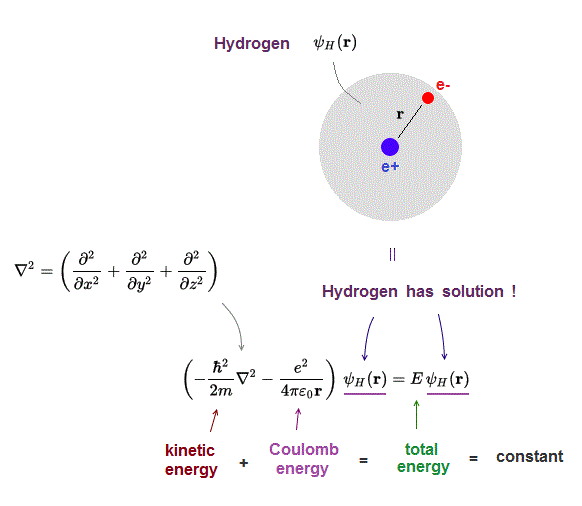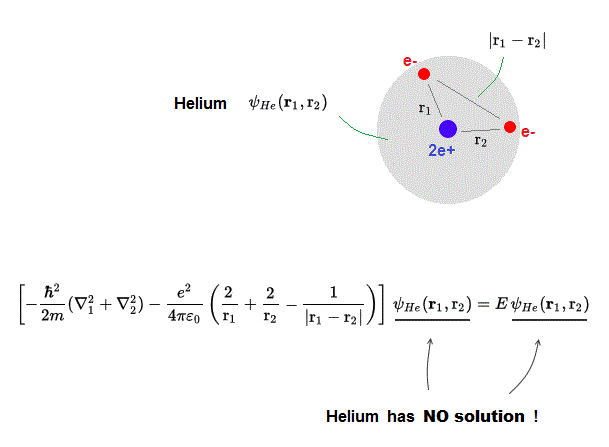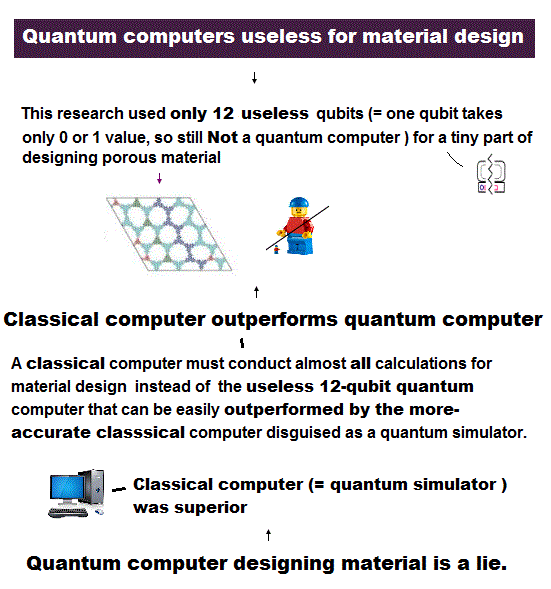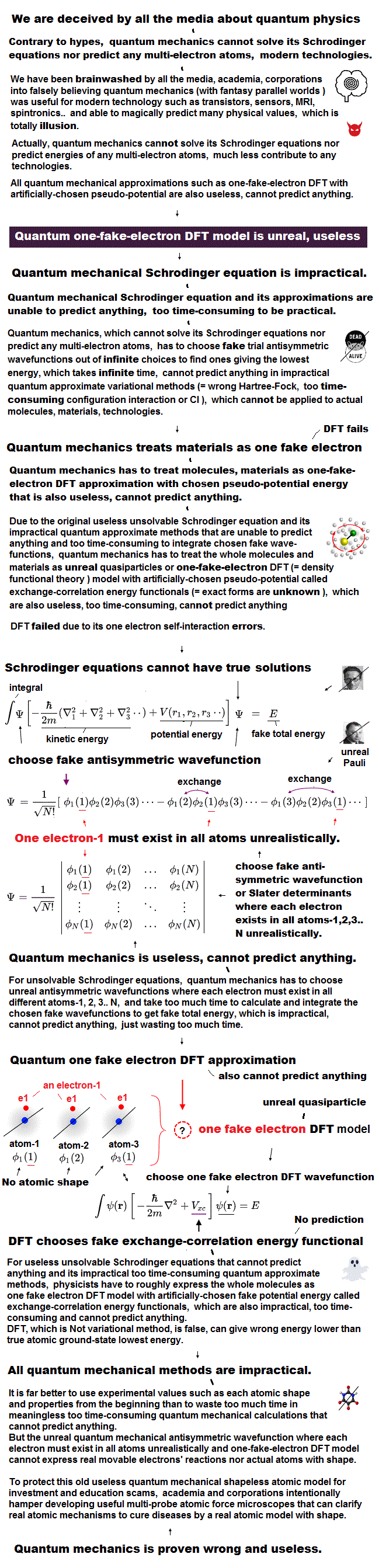﻿ Density functional theory (= DFT ) is of NO practical use

# Density functional theory (= DFT ) is useless

## Schrödinger equation for hydrogen can be solved.

### [ One-electron hydrogen atom is the only solvable Schrödinger equation ! ]

(Fig.1)  Schrödinger equation for hydrogen atom = a proton + an electronOnly Schrödinger equation for one-electron hydrogen atom can be solved and has exact solution.

Schrodinger equation gives the constant total energy equal to the sum of kinetic and potential energies.

## Two-electron Helium has NO solution !

### [ Schrödinger equation for Helium atom cannot be solved ! ]

(Fig.2)  ↓ Schrödinger equation Helium = two electrons (e-) + one nucleus (2e+)Schrödinger equation for multi-electron atoms such as Helium cannot be solved !  Helium atom consists of two electrons ( e- ) and one nucleus ( 2e+ ).

Helium's total energy E should be the sum of kinetic energy and Coulomb potential energy.

r1 ( r2 ) is the distance between the nucleus and electron 1 ( electron 2 ).
|r1 - r2| is the distance between two electrons

## Density functional theory (= DFT ) is useless.

### [ DFT depends on "fake" potential for which you can choose any form. ]

(Fig.3)  You can choose any "fake potential" out of infinite choices in DFT !Schrödinger equation cannot give true solution.  So to handle multi-electron atoms, they invented density functional theory (= DFT ).

This DFT calculation method is used in the applied fields such as condensed matter and protein interaction only for publishing paper ( NOT for practical use ! )

DFT artificially changes "multi-electron Schrödinger equation" into one-electron density form and depends on "fake potential".

This fake potential is supposed to describe "electron-electron interaction", but its functional form (= Vxc ) is unknown.

You can choose any forms for this functional Vxc (= called "exchange-correlation" ) out of infinite choices.  So DFT has NO ability to predict any physical values.

## Coulomb potential → fake potential = DFT

### [ Fake potential is chosen to fit experiment = meaningless. ]

(Fig.4)  Multi-electron Couloum energy → fake potential in DFT, so meagninelssIn condensed matter and solids including almost infinite atoms, computing by Schrödinger equation is far more impossible.

So physicists forcedly replaced multi-electron Schrödinger equation by one-electron DFT form which includes artificial fake potential.

There are NO restrictions in choosing this fake potential.  You can pick up any form of fake potential Vxc out of infinite choices !

No matter what new "fake potential" is artificially invented, someone will always find a case where it fails ( this p.17 ).

DFT has NO ablity to predict any physical phenomena, so useless and meaningless.  DFT is basically an "empirical" method ( this p.23 )

## The current science has stopped its progress !

### [ Physicists still keep using useless computing methods forever. ]

(Fig.5)  Now, almost all applied physics depends on useless DFT method.One-electron hydrogen atom is the only one in which Schrödinger equation can be applied.  Almost all other applied physics depends on DFT.

This is the reason why the current condensed matter physics is useless, depending only on unreal quasipalrticles.

Physicists use this DFT method only for publishing their papers in science journals, NOT for practical use !2017/2/10 updated. Feel free to link to this site.